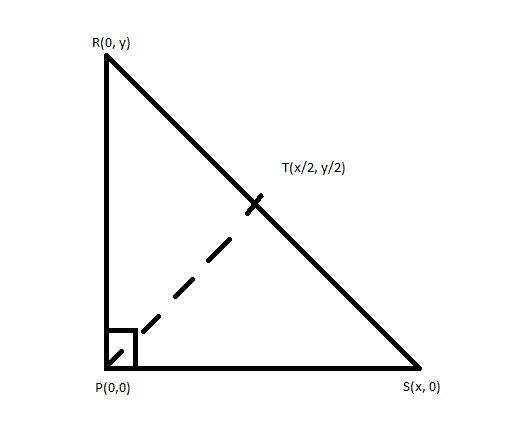# Use vectors and the dot product to prove the midpoint

## Homework Statement

[/B]
Use vectors and the dot product to prove that the midpoint of the hypotenuse of a right triangle is equidistant to all three vertices.

## Homework Equations

[/B]
I know the dot product is A⋅B = |A||B|cosΘ ...... or .... A1B1 + A2B2 + A3B3 ... + AnBn

I know the magnitude of a vector is its length, and is given by√(x^2 + y^2) I have omitted the z^2 since I want to solve this in R^2.

## The Attempt at a Solution

[/B]
I figure doing this in R^2 is easier, so I drew a right triangle with points P(0,0), R(0, y), S(x, 0)

I know the midpoint would be given by T = (x/2, y/2)

I constructed vectors using these points:

PS = <x, 0>
PR = <0, y>
TS = <x/2, -y/2>
RT = <x/2, -y/2>
PT = <x/2, y/2>

From this I can show that the magnitude of TS, RT, and PT are all the same, thus their distances are the same. I can also use this to show that TS = RT. I can then take the dot product of TS and RT and work it down to show the angle between them is 0 (so cosΘ = 1) and thus they are the same vector.

What I cannot do for the life of me is use the dot product to prove that PT has the same magnitude as the other two vectors, TS and RT. I just can't figure it out. I've tried finding ways to express PT as other vectors but everything I've tried has failed. I am tearing my hair out!

Here is a diagram of how I am trying to layout my triangle:I would greatly appreciate any insight into this very troubling problem. :(

Last edited:

Stephen Tashi
I don't know what the exercise considers "using the dot product". Computing the magnitude of a vector can be regarded as using the dot product. $|A| = \sqrt{ A \cdot A}$.

There is a vector version of the law of cosines:

If $C = A + B$ then $|C|^2 = |A|^2 + |B|^2 + 2( A \cdot B)$.

If you set $C = A - B$ then it looks like the law of cosines from trigonometry. $|C|^2 = |A|^2 + |B|^2 - 2( A \cdot B)$.

Whether it is acceptable to use the law of cosines for vectors depends on what's been covered in your course.

I don't know what the exercise considers "using the dot product". Computing the magnitude of a vector can be regarded as using the dot product. $|A| = \sqrt{ A \cdot A}$.

There is a vector version of the law of cosines:

If $C = A + B$ then $|C|^2 = |A|^2 + |B|^2 + 2( A \cdot B)$.

If you set $C = A - B$ then it looks like the law of cosines from trigonometry. $|C|^2 = |A|^2 + |B|^2 - 2( A \cdot B)$.

Whether it is acceptable to use the law of cosines for vectors depends on what's been covered in your course.

Hey, thank you for your reply. I went about it a little differently than I originally posted and was able to prove it. Thanks for mentioning that a vector's magnitude is equal to the square root of that vector dotted by itself. Using that idea, I was able to prove that the distance to every vertex was the same. I completely forgot about that property, and it turns out it's quite useful, haha. :)